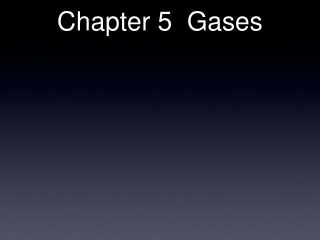DownloadDownload PresentationChapter 5 Gases

# Chapter 5 Gases

Télécharger la présentation## Chapter 5 Gases

- - - - - - - - - - - - - - - - - - - - - - - - - - - E N D - - - - - - - - - - - - - - - - - - - - - - - - - - -
##### Presentation Transcript

1. Chapter 5 Gases

2. Chapter 5 Gases

3. WHY?

4. Sketch of ModernBalloons

5. WHY?

6. Mathematics • V/T = constant

7. 1.00 PSI

8. 8.00 PSI

9. 8.00 PSI WHY?

10. Mathematics • PV = constant

11. Mathematics • V/n = constant • (n = moles)

12. Moles

13. 273 1.00 K ATM

14. 804 9.79 K ATM

15. (atm)

16. Mathematics • P/T= constant

17. MathematicsSummary • V/n = constant • V/T = constant • P/T = constant • PV = constant

18. MathematicsSummary • V/nT = constant • P/T = constant • PV/nT = constant

19. MathematicsSummary • PV/nT = constant • PV = nTconstant • constant = R • PV = nRT Ideal Gas Law

20. Conditions of Use • PV = nRT Ideal Gas Law • P units must be atm’s • V units must be liters • T must be in Kelvin

21. Gas Law ResourcesPressures equivalent to the standard 1.00 ATM • 28.3 ft. H2O • 29.92 in. Hg • 76.0 cm Hg • 760.0 mm Hg • 760.0 torr • 14.7 psi. • 1.013 bar • 1.013 x 105 Pa • 101.3 kPa • (1 Pa = 1 Nt/M2)

22. Practice • Fill out the following chart. • mm Hg. atm. kPa. bar • 1215 . • 0.714 . • 143 . • 0.904 .

23. Gas Law ResourcesTemperature - must be absolute - never negative • Temperature must be in Kelvin • K = oC + 273

24. Practice • Calculate the volume of 1.0 mole of a gas at Standard Temperature and Pressure.

25. Practice • Fill out the following chart for C4H10 • Press. Vol. Temp. Moles Grams • 1.75 L 19 oC 1.66 . • 0.895 atm 6.0 oC 14.0 . • 433 mm Hg 92.4 mL 0.395 . • 1.74 bar 8.66 L 310 K .

26. Experiment

27. Experiment

28. Experiment 4 Fe + 3 O2→ 2 Fe2O3 Fe + N2→ NR

29. Interpretation • Air contains both N2 and O2 • Each gas has its own independent pressure. • “The sum of all individual gas pressures in a gas mixture is equal to the total pressure” DALTON • Dalton’s Law of Partial Pressures

30. (go to video)

31. KMT • Kinetic Molecular Theory of gases • Gases consist of very tiny particles in continuous random motion. • Particle collisions are elastic(no energy lost). • Particle volume is negligible. • Particle interaction is negligible. • molecular speed causes both pressure and volume of gases

32. Graham’s Law • Often called Grahams’ Law of Diffusion - in error • Diffusion - movement of gas down an open tube • complex and influenced by many factors. • Effusion - movement of gas through small holes. • Graham’s Law of Effusion of Gases • “At a given temperature and pressure, gaseous effusion rate in moles per time unit, is inversely proportional to the square root of the molar mass of the gases”. • effusion rate of B = √mm B • effusion rate of A √mm A

33. Ideal vs Real • Review KMT. Obvious non-realities. • Some gas molecules are NOT tiny. • Many gas collisions DO lose energy • Gas molecules HAVE a noticeable volume • Some molecules have SIGNIFICANT interaction.